nextnano.com  GUI: nextnanomat  Tool: nextnano++  Tool: nextnano³  Tool: nextnano.QCL  Download | Search | Copyright | News | Publications  * password protected nextnano³ software1D bulk k.p dispersion in GaAs

# nextnano3 - Tutorial

## k.p dispersion in bulk GaAs (strained / unstrained)

Author: Stefan Birner

If you want to obtain the input files that are used within this tutorial, please contact stefan.birner@nextnano.de.
```-> bulk_kp_dispersion_GaAs_nn3.in          / *_nnp.in          - ```input file for nextnano3 / nextnano++ software``` -> bulk_kp_dispersion_GaAs_nn3_3D.in                           - ```input file for nextnano3``` -> bulk_kp_dispersion_GaAs_nn3_strained.in / *_nnp_strained.in - ```input file for nextnano3 / nextnano++ software

## Band structure of bulk GaAs

• We want to calculate the dispersion E(k) from |k|=0 nm-1 to |k|=1.0 nm-1 along the following directions in k space:
-  to 
-  to 
We compare 6-band and 8-band k.p theory results.
• We calculate E(k) for bulk GaAs at a temperature of 300 K.

## Bulk dispersion along  and along 

• ``` \$output-kp-data  destination-directory    = kp/  bulk-kp-dispersion       = yes  grid-position            = 5d0                      ! ```in units of` [nm]````  !----------------------------------------  ! Dispersion along  direction  ! Dispersion along  direction  ! maximum |k| vector = 1.0 [1/nm]  !----------------------------------------  k-direction-from-k-point = 0d0  0.7071d0  0.7071d0  ! ```k-direction and range for dispersion plot``` [1/nm]  k-direction-to-k-point   = 0d0  0d0       1.0d0     ! ```k-direction and range for dispersion plot``` [1/nm]  ! ```The dispersion is calculated from the k point '`k-direction-from-k-point`' to Gamma, and then from the Gamma point to '`k-direction-to-k-point`'.```  number-of-k-points       = 100                      ! ```number of k points to be calculated (resolution)
``` shift-holes-to-zero      = yes                      ! 'yes' ```or``` 'no' \$end_output-kp-data```
• We calculate the pure bulk dispersion at` grid-position=5d0`, i.e. for the material located at the grid point at 5 nm. In our case this is GaAs but it could be any strained alloy. In the latter case, the k.p Bir-Pikus strain Hamiltonian will be diagonalized.
The grid point at` grid-position `must be located inside a quantum cluster.
`shift-holes-to-zero = yes `forces the top of the valence band to be located at 0 eV.
How often the bulk k.p Hamiltonian should be solved can be specified via` number-of-k-points`. To increase the resolution, just increase this number.
• We use two direction is k space, i.e. from  to  and from  to . In the latter case the maximum value of |k| is SQRT(0.7071² + 0.7071²) = 1.0.
Note that for values of |k| larger than 1.0 nm`-`1, k.p theory might not be a good approximation any more.Start the calculation.
The results can be found in` kp_bulk/bulk_8x8kp_dispersion_as_in_inputfile_kxkykz_000_kxkykz.dat`.

## Step 3: Plotting E(k)

• Here we visualize the results. The final figure will look like this: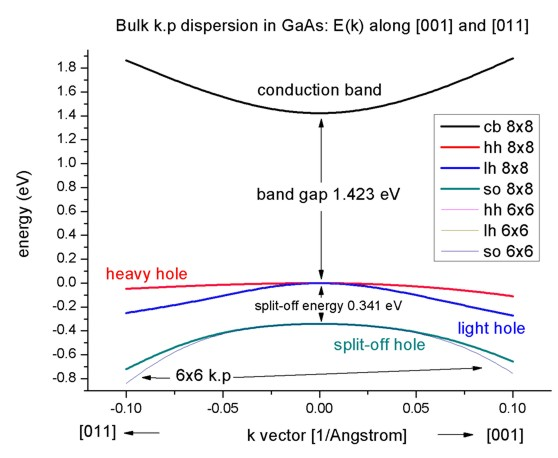The split-off energy of 0.341 eV is identical to the split-off energy as defined in the database:
```  6x6-kp-parameters = ... 0.341d0 !  [eV] ```
• If one zooms into the holes and compares 6-band vs. 8-band k.p, one can see that 6-band and 8-band coincide for |k| < 1.0 nm`-`1 for the heavy and light hole but differ for the split-off hole at larger |k| values.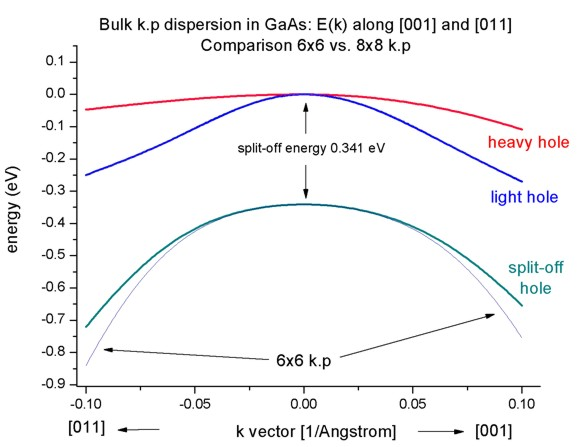To switch between 6-band and 8-band k.p one only has to change this entry in the input file:
``` \$quantum-model-holes  ...  model-name = 8x8kp ! ```for 8-band k.p```             = 6x6kp ! ```for 6-band k.p

## 8-band k.p vs. effective-mass approximation

• Now we want to compare the 8-band k.p dispersion with the effective-mass approximation. The effective mass approximation is a simple parabolic dispersion which is isotropic (i.e. no dependence on the k vector direction). For low values of k (|k| < 0.4 nm`-`1) it is in good agreement with k.p theory.
The output data can be find here:
` kp_bulk/bulk_sg_dispersion.dat`.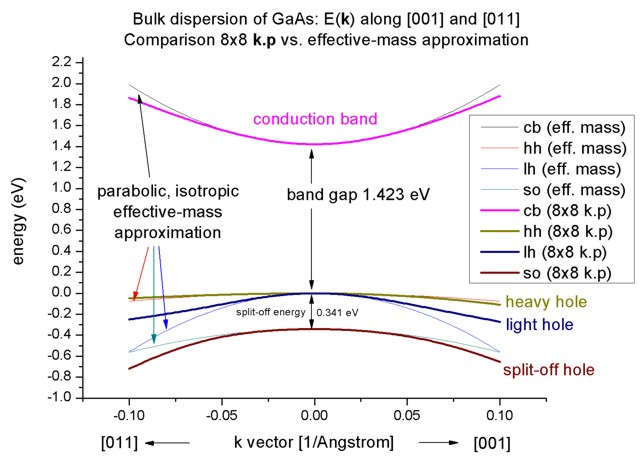## Band structure of strained GaAs

• Now we perform these calculations again for GaAs that is strained with respect to In0.2Ga0.8As. The InGaAs lattice constant is larger than the GaAs one, thus GaAs is strained tensilely.
• The changes that we have to make in the input file are the following:

``` \$simulation-flow-control  ...  strain-calculation  = homogeneous-strain \$end_simulation-flow-control ```
``` \$domain-coordinates  ...  pseudomorphic-on    = In(x)Ga(1-x)As  alloy-concentration = 0.20d0 \$end_domain-coordinates ```As substrate material we take In0.2Ga0.8As and assume that GaAs is strained pseudomorphically (`homogeneous-strain`) with respect to this substrate, i.e. GaAs is subject to a biaxial strain.
• Due to the positive hydrostatic strain (i.e. increase in volume or negative hydrostatic pressure) we obtain a reduced band gap with respect to the unstrained GaAs.
Furthermore, the degeneracy of the heavy and light hole at k=0 is lifted.
Now, the anisotropy of the holes along the different directions  and  is very pronounced. There is even a band anti-crossing along . (Actually, the anti-crossing looks like a "crossing" of the bands but if one zooms into it (not shown in this tutorial), one can easily see it.)
Note: If biaxial strain is present, the directions along x, y or z are not equivalent any more. This means that the dispersion is also different in these directions (, , ).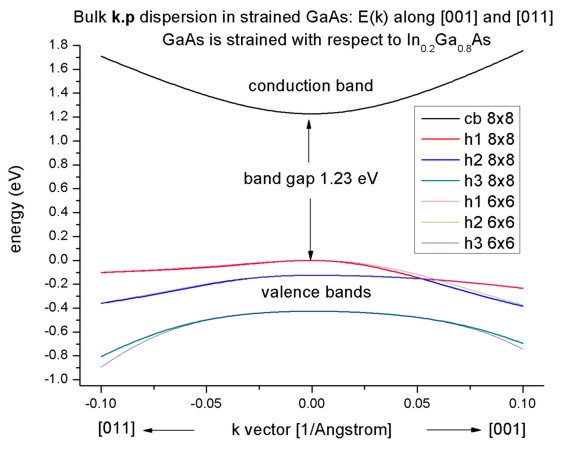• If one zooms into the holes and compares 6-band vs. 8-band k.p, one can see that the agreement between heavy and light holes is not as good as in the unstrained case where 6-band and 8-band k.p lead to almost identical dispersions.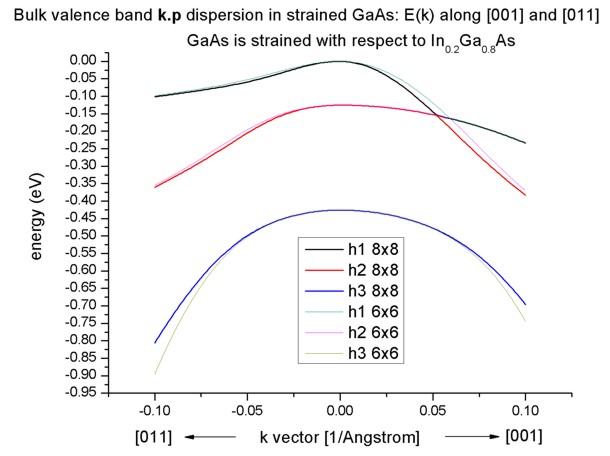Note that in the strained case, the effective-mass approximation is very poor.

### Analysis of eigenvectors

(preliminary)

Using``` schroedinger-kp-basis = Voon-Willatzen--Bastard--Foreman ```(box-integration) one obtains the following output for the eigenvectors at the Gamma point, k = (kx,ky,kz) = 0.

The square of the spinor is contained in this file:``` bulk_8x8kp_dispersion_eigenvectors_squared_000.dat ```Example: The` X_up `component contains a complex number. Here, we output the square of` X_up`. This file then gives us information on the strength of the coupling of the mixed states.
This file is easier to analyze then the file containing the complex numbers (`bulk_8x8kp_dispersion_eigenvectors_000.dat`).

``` eigenvalue  S+     S-     HH     LH     LH     LH     SO     SO  1           0      1.0    0      0      0      0      0      0  2           1.0    0      0      0      0      0      0      0  3           0      0      0      1.0    0      0      0      0  4           0      0      0      0      1.0    0      0      0  5           0      0      0      0      0      1.0    0      0  6           0      0      1.0    0      0      0      0      0  7           0      0      0      0      0      0      0      1.0  8           0      0      0      0      0      0      1.0    0```

``` eigenvalue  S+          S-          X+          Y+          Z+          X-          Y-          Z-  1           1.0         0           0           0           0           0           0           0  2           0           1.0         0           0           0           0           0           0  3           0           0           0           0           0.5         0.5         0           0  4           0           0           0           0           0.166       0.166       0.666       0  5           0           0           0.5         0           0           0           0           0.5  6           0           0           0.166       0.666       0           0           0           0.166  7           0           0           0           0           0.333       0.333       0.333       0  8           0           0           0.333       0.333       0           0           0           0.333  ```

`+`: spin up,` -`: spin down

The electron eigenstates are 2-fold degenerate, i.e. have the same energy, and are decoupled from the holes.

• ```1: S_down 2: S_up```

The hole eigenstates are 4-fold (heavy and light holes) and 2-fold degenerate (split-off holes).

• ```3: |3/2, 3/2> hh spin up      1/SQRT(2) | ( X + iY ) up   > 4: |3/2, 1/2> lh              1/SQRT(6) | ( X + iY ) down >  -  SQRT(2/3) | Z up > 5: |3/2,-1/2> lh             -1/SQRT(6) | ( X - iY ) up   >  -  SQRT(2/3) | Z down > 6: |3/2,-3/2> hh spin down    1/SQRT(2) | ( X - iY ) down > 7: |1/2, 1/2> s/o split       1/SQRT(3) | ( X + iY ) down >  +  1/SQRT(3) | Z up > 8: |1/2,-1/2> s/o split      -1/SQRT(3) | ( X - iY ) up   >  +  1/SQRT(3) | Z down > ```
• `up`: spin up,` down`: spin down``` 1/SQRT(2) = 0.707  ==>  1/SQRT(2)^2 = 0.5 1/SQRT(3) = 0.577  ==>  1/SQRT(3)^2 = 0.333 1/SQRT(6) = 0.408  ==>  1/SQRT(6)^2 = 0.166```

• Please help us to improve our tutorial! Send comments to``` support [at] nextnano.com```.